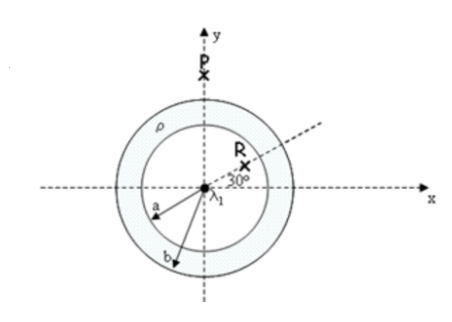# Problem: An infinite line of charge with linear density λ1 = 8.8 µC/m is positioned along the axis of a thick insulating shell of inner radius a = 2 cm and outer radius b = 4.1 cm. The insulating shell is uniformly charged with a volume density of ρ = 688 µC/m3.1) What is Ey(P), the value of the y-component of the electric field at point P, located a distance 7.4 cm along the y-axis from the line of charge? Given that linear charge density of the insulating shell, λ2 = 2.769 × 10-6 C/m3.2) What is Ex(R), the value of the x-component of the electric field at point R, located a distance 1 cm along a line that makes an angle of 30o with the x-axis?

###### FREE Expert Solution

1)

Ey(P) = (λ1 + λ2)/(2πrε0)

86% (247 ratings)###### Problem Details

An infinite line of charge with linear density λ1 = 8.8 µC/m is positioned along the axis of a thick insulating shell of inner radius a = 2 cm and outer radius b = 4.1 cm. The insulating shell is uniformly charged with a volume density of ρ = 688 µC/m3.1) What is Ey(P), the value of the y-component of the electric field at point P, located a distance 7.4 cm along the y-axis from the line of charge? Given that linear charge density of the insulating shell, λ2 = 2.769 × 10-6 C/m3.

2) What is Ex(R), the value of the x-component of the electric field at point R, located a distance 1 cm along a line that makes an angle of 30o with the x-axis?